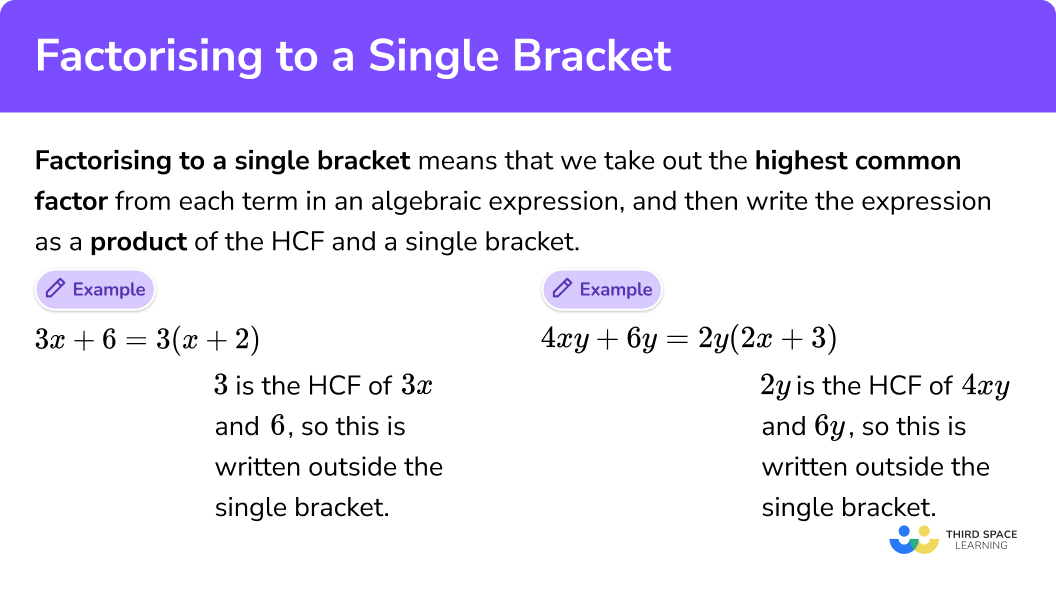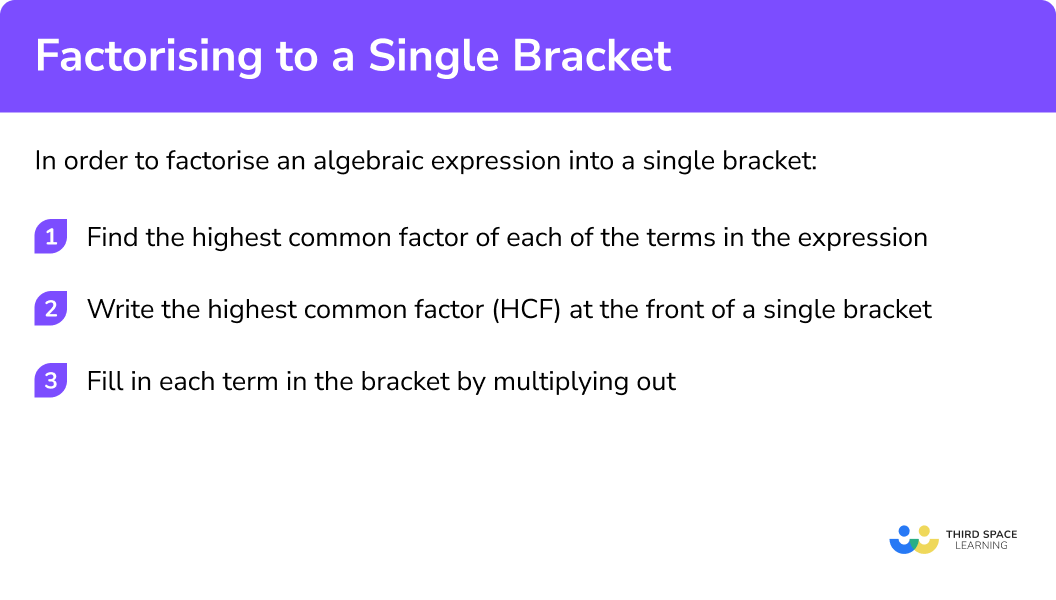# Factorising Single Brackets

Here we will learn about factorising to single brackets by finding the highest common factor.

There are also factorising to single brackets worksheets based on Edexcel, AQA and OCR exam questions, along with further guidance on where to go next if you’re still stuck.

### What is factorising single brackets?## How to factorise into single brackets

In order to factorise an algebraic expression into a single bracket:

1. Find the highest common factor of each of the terms in the expression.
2. Write the highest common factor (HCF) at the front of a single bracket
3. Fill in each term in the bracket by multiplying out.

### Explain how to factorise into single brackets in 3 stepsWe can factorise lots of different types of expressions into single brackets including some quadratics like x2 + 5 or 3x2 – 5x.

For quadratic expressions of the form x2 + bx + c or ax2 + bx + c we will need to factorise into double brackets – you can learn all about this in the factorising quadratics lesson.

Check out our main factorising lesson for a complete summary of all the different ways we can factorise expressions, and then explore our other factorising lessons for detailed step-by-step guides and worksheets on each type.

## Factorising single brackets examples

### Example 1: variable in just one term

Fully factorise:

$\color{#00BC89}3x \color{#7C4DFF}{+6}$

1Find the highest common factor (HCF) of the numbers 3 (the coefficient of x) and 6 (the constant).

Factors of 3:
1, 3

Factors of 6:
1, 6
2, 3

Top tip:
Writing the factor pairs makes it easier to list all the factors

The highest common factor (HCF) of 3x and 6 is 3

2Write the highest common factor (HCF) at the front of the single bracket.

$\color{#FF9100}3(\qquad+\qquad)$

3Fill in each term in the bracket by multiplying out.

What do I need to multiply 3 by to give me 3x?

$\color{#FF9100}3\times \color{#62F030}x = \color{#00bc89}3x$

What do I need to multiply 3 by to give me 6?

$\color{#FF9100}3\times \color{#92009E}2 = \color{#7C4DFF}6$

$\color{#FF9100}3(\color{#62F030}x+\color{#92009E}2)$

We can check the answer by multiplying out the bracket!

$3(x+2)=3x+6$

### Example 2: variable in just one term

Fully factorise:

$\color{#7C4DFF}{14}\color{#00BC89}{-7}y$

Factors of 14:
1, 14
2, 7

Factors of 7:
1, 7

The highest common factor (HCF) of 14 and 7y is 7

$\color{#FF9100}7(\qquad-\qquad)$

What do I need to multiply 7 by to give me 14?

$\color{#FF9100}7\times \color{#62F030}2 = \color{#7C4DFF}{14}$

What do I need to multiply 7 by to give me 7y?

$\color{#FF9100}7\times \color{#92009E}y = \color{#00bc89}7y$

$\color{#FF9100}7(\color{#62F030}2-\color{#92009E}y)$

(don’t forget to keep the – here)

We can check the answer by multiplying out the bracket!

$7(2-y)=14-7y$

### Example 3: variable in two terms

Fully factorise:

$\color{#FE47EC}8x^{2}\color{#00BC89}{+12}x$

Factors of 8:
1, 8
2, 4

Factors of 12:
1, 12
2, 6
3, 4

Find the highest common factor (HCF) of the variables x2 and x

x2 = x x

x = x

The highest common factor (HCF) of 8x2 and 12x is 4x

$\color{#FF9100}{4x}(\qquad+\qquad)$

What do I need to multiply 4x by to give me 8x2 ?

$\color{#FF9100}{4x} \times \color{#62F030}{2x} = \color{#FE47EC}8x^2$

What do I need to multiply 4x by give me 12x?

$\color{#FF9100}{4x} \times \color{#92009E}3 = \color{#00BC89}{12}x$

$\color{#FF9100}{4x}(\color{#62F030}{2x} + \color{#92009E}3)$

We can check the answer by multiplying out the bracket!

4x(2x + 3) = 8x2 + 12x

### Example 4: variable in two terms

Fully factorise:

$\color{#FE47EC}{15}y^{2}\color{#0EE2EF}{-10}xy$

Factors of 15:
1, 15
3, 5

Factors of 10:
1, 10
2, 5

Find the highest common factor (HCF) of the letters y2 and xy

y2 = y ✕ y

xy = x ✕ y

The highest common factor (HCF) of 15y2 and 10xy is 5y

$\color{#FF9100}{5y}(\qquad-\qquad)$

What do I need to multiply 5y by to give me 15y2?

$\color{#FF9100}{5y} \times \color{#62F030}{3y} = \color{#FE47EC}{15}y^2$

What do I need to multiply 5y by to give me 10xy?

$\color{#FF9100}{5y} \times \color{#92009E}{2x} = \color{#0EE2EF}{10}xy$

$\color{#FF9100}{5y}(\color{#62F030}{3y}\color{#92009E}{-2x})$

We can check the answer by multiplying out the bracket!

$5y(3y-2x)=15y^{2}-10xy$

### Example 5: variable in two out of three terms

Fully factorise:

$\color{#00BC89}{6}x \color{#00BC89}{+2}y \color{#7C4DFF}{- 12}$

Factors of 6:
1, 6
2, 3

Factors of 2:
1, 2

Factors of 12:
1, 12
2, 6
3,4

The highest common factor (HCF) of 6 and 2 and 12 is 2

$\color{#FF9100}2(\qquad+\qquad-\qquad)$

What do I need to multiply 2 by to give me 6x?

$\color{#FF9100}2 \times \color{#62F030}{3x} = \color{#00bc89}6x$

What do I need to multiply 2 by to give me 2y?

$\color{#FF9100}2 \times \color{#92009E}y = \color{#00bc89}2y$

What do I need to multiply 2 by to give me 12?

$\color{#FF9100}2 \times \color{#F6C929}6 = \color{#7C4DFF}{12}$

$\color{#FF9100}2(\color{#62F030}{3x} \color{#92009E}{+y} \color{#F6C929}{-6})$

Don’t forget to keep the – here!

We can check the answer by multiplying out the bracket!

$2(3x+y-6)=6x+2y-12$

### Example 6: variable in two out of three terms

Fully factorise:

$\color{#0EE2EF}{12}xy\color{#FE47EC}{-4}x^{2}y\color{#FE47EC}{+8}xy^{2}$

Factors of 12:
1, 12
2, 6
3,4

Factors of 4:
1, 4
2, 2

Factors of 8:
1, 8
2, 4

Find the highest common factor (HCF) of the letters x2 and x2y and xy2

xy = xy

x2y = x ✕ xy

xy2 = xy y

The highest common factor (HCF) of 12x2 and 4x2y and 8xy2 is 4xy

$\color{#FF9100}4xy(\qquad-\qquad+\qquad)$

What do I need to multiply 4xy by to give me 12xy?

$\color{#FF9100}{4xy} \times \color{#62F030}3 = \color{#0EE2EF}{12}xy$

What do I need to multiply 4xy by to give me 4x2y?

$\color{#FF9100}{4xy} \times \color{#92009E}x = \color{#FE47EC}4x^2y$

What do I need to multiply 4xy by to give me 8xy2?

$\color{#FF9100}{4xy} \times \color{#F6C929}{2y} = \color{#FE47EC}8xy^2$

$\color{#FF9100}{4xy}( \color{#62F030}3\color{#92009E}{-x}\color{#F6C929}{+2y})$

Don’t forget to keep the – here!

We can check the answer by multiplying out the bracket!

$4xy(3-x+2y)=12xy-4x^{2}y+8xy^{2}$

## Common misconceptions

These are some of the common misconceptions around factorising into single brackets

• We must fully factorise

12x2 – 6x = 2(6x2 – 3x)

Here we have factorised the expression, however it is not fully factorised because 2 is not the highest common factor.

6x is the highest common factor, so this is the correct final answer:

12x2 – 6x = 6x(2x – 1)

• 12x2 – 6x

Even though this a quadratic expression we still factorise it to give a single bracket because it is not in the form ax2 + bx + c

## Practice factorising single brackets questions

1. Fully factorise: 5x+10

5(x+10)5x(x+2)5(x+2)x(5+10)The highest common factor of 5 and 10 is 5 . We can divide each term in the original expression by 5 , which means the bracket must contain (x+2) .

2. Fully factorise: 8-2y

2(4-y)2(4+y)2y(4-y)8(1-2y)The highest common factor of 8 and 2 is 2 . We can divide each term in the original expression by 2 ; the bracket must contain a minus sign just like the original expression.

3. Fully factorise: 18x^{2}-12x

3x(6x-4)6(3x^{2}-2x)3(6x^{2}-4x)6x(3x-2)The highest common factor of 18x^{2} and 12x is 6x . We can divide each term by 6x , which means the bracket must contain (3x-2) .

4. Fully factorise: 20y^{2}+16xy

4y(5y-4x)y(20y-16x)2y(10y-8x)4xy(5y-4x)The highest common factor of 20y^{2} and 16xy is 4y . We can divide each term by 4y , which means the bracket must contain (5y-4x) .

5. Fully factorise: 18-6y+15x

3xy(6-2y+5x)3(6-2y+5x)18(1-2y+5x)3(6+2y+5x)The highest common factor of 18,6y and 15x is 3 . We can divide each term by 3 , which means the bracket must contain (6-2y+5x) .

6. Fully factorise: 12y-9x^{2}y+6y^{2}

12y(1-3x^{2}+2y)3y(4+3x^{2}+2y)3y(4-3x^{2}+2y)3y(4-3x^{2}-2y)The highest common factor of 12y, 9x^{2}y and 6y^{2} is 3y . We can divide each term by 3y , which means the bracket must contain (4-3x^{2}+2y) .

## Factorising single brackets GCSE questions

1. Factorise: 5x-20

5(x-4)

(1 mark)

2. Factorise fully: 8x2 + 12xy

4x(2x+3y)

(2 marks)

3. Factorise: x2 + 8x

x(x+8)

(1 mark)

## Learning checklist

• Manipulate algebraic expressions by taking out common factors to factorise into a single bracket
• Factorise quadratic expressions of the form x2 + bx + c
• Factorise quadratic expressions of the form of the difference of two squares
• Factorising quadratic expressions of the form ax2 + bx + c (H)

## Still stuck?

Prepare your KS4 students for maths GCSEs success with Third Space Learning. Weekly online one to one GCSE maths revision lessons delivered by expert maths tutors.

Find out more about our GCSE maths tuition programme.